# 评估机器学习模型

## 2022年10月

Posted by franztao on October 1, 2022

## intuition

• 清楚优先考虑的指标
• 注意不要过度优化任何一个指标，因为这可能意味着你正在妥协其他指标
# Metrics
metrics = {"overall": {}, "class": {}}

 # Data to evaluate
other_index = label_encoder.class_to_index["other"]
y_prob = model.predict_proba(X_test)
y_pred = custom_predict(y_prob=y_prob, threshold=threshold, index=other_index)


## 粗粒度

from sklearn.metrics import precision_recall_fscore_support
# Overall metrics
overall_metrics = precision_recall_fscore_support(y_test, y_pred, average="weighted")
metrics["overall"]["precision"] = overall_metrics
metrics["overall"]["recall"] = overall_metrics
metrics["overall"]["f1"] = overall_metrics
metrics["overall"]["num_samples"] = np.float64(len(y_test))
print (json.dumps(metrics["overall"], indent=4))

{
“precision”：0.8990934378802025，
“recall”：0.8194444444444444，
“f1”：0.838280325954406，
“num_samples”：144.0
}


note

• None：针对每个唯一类计算指标。
• binary：用于pos_label指定 的二进制分类任务。
• micro: 使用全局 TP、FP 和 FN 计算指标。
• macro：在不考虑类不平衡的情况下平均每类指标。
• weighted：通过考虑类不平衡来平均的每类指标。
• samples：指标是在每个样本级别计算的。

## 细粒度

from collections import OrderedDict
# Per-class metrics
class_metrics = precision_recall_fscore_support(y_test, y_pred, average=None)
for i, _class in enumerate(label_encoder.classes):
metrics["class"][_class] = {
"precision": class_metrics[i],
"recall": class_metrics[i],
"f1": class_metrics[i],
"num_samples": np.float64(class_metrics[i]),
}

# Metrics for a specific class
tag = "natural-language-processing"
print (json.dumps(metrics["class"][tag], indent=2))

{
"precision": 0.9803921568627451,
"recall": 0.8620689655172413,
"f1": 0.9174311926605505,
"num_samples": 58.0
}

# Sorted tags
sorted_tags_by_f1 = OrderedDict(sorted(
metrics["class"].items(), key=lambda tag: tag["f1"], reverse=True))
for item in sorted_tags_by_f1.items():
print (json.dumps(item, indent=2))

[
"natural-language-processing",
{
"precision": 0.9803921568627451,
"recall": 0.8620689655172413,
"f1": 0.9174311926605505,
"num_samples": 58.0
}
]
[
"mlops",
{
"precision": 0.9090909090909091,
"recall": 0.8333333333333334,
"f1": 0.8695652173913043,
"num_samples": 12.0
}
]
[
"computer-vision",
{
"precision": 0.975,
"recall": 0.7222222222222222,
"f1": 0.8297872340425532,
"num_samples": 54.0
}
]
[
"other",
{
"precision": 0.4523809523809524,
"recall": 0.95,
"f1": 0.6129032258064516,
"num_samples": 20.0
}
]


## 混淆矩阵

• 真阳性（TP）：了解模型在哪些方面表现良好。
• 误报（FP）：潜在地识别可能需要重新标记的样本。
• 假阴性 (FN)：识别模型性能较差的区域，以便稍后进行过采样。

# TP, FP, FN samples
tag = "mlops"
index = label_encoder.class_to_index[tag]
tp, fp, fn = [], [], []
for i, true in enumerate(y_test):
pred = y_pred[i]
if index==true==pred:
tp.append(i)
elif index!=true and index==pred:
fp.append(i)
elif index==true and index!=pred:
fn.append(i)

print (tp)
print (fp)
print (fn)

[1, 3, 4, 41, 47, 77, 94, 127, 138]
[14, 88]
[30, 71, 106]

index = tp
print (X_test_raw[index])
print (f"true: {label_encoder.decode([y_test[index]])}")
print (f"pred: {label_encoder.decode([y_pred[index]])}")

pytest pytest framework makes easy write small tests yet scales support complex functional testing
true: mlops
pred: mlops

# Samples
num_samples = 3
cm = [(tp, "True positives"), (fp, "False positives"), (fn, "False negatives")]
for item in cm:
if len(item):
print (f"\n=== {item} ===")
for index in item[:num_samples]:
print (f"  {X_test_raw[index]}")
print (f"    true: {label_encoder.decode([y_test[index]])}")
print (f"    pred: {label_encoder.decode([y_pred[index]])}\n")

=== True positives ===
pytest pytest framework makes easy write small tests yet scales support complex functional testing
true: mlops
pred: mlops

test machine learning code systems minimal examples testing machine learning correct implementation expected learned behavior model performance
true: mlops
pred: mlops

continuous machine learning cml cml helps organize mlops infrastructure top traditional software engineering stack instead creating separate ai platforms
true: mlops
pred: mlops

=== False positives ===
paint machine learning web app allows create landscape painting style bob ross using deep learning model served using spell model server
true: computer-vision
pred: mlops

=== False negatives ===
hidden technical debt machine learning systems using software engineering framework technical debt find common incur massive ongoing maintenance costs real world ml systems
true: mlops
pred: other

neptune ai lightweight experiment management tool fits workflow
true: mlops
pred: other


TIP

## Confident learning

• 分类的

• 预测不正确（也表示TN、FP、FN）
• 正确类别的置信度分数低于阈值
• 错误类别的置信度分数高于阈值
• 前 N 个样本的置信度得分的标准差较低
• 使用不同参数的同一模型的不同预测
• 连续的

• 预测值和真实值之间的差异高于某个百分比
# y
y_prob = model.predict_proba(X_test)
print (np.shape(y_test))
print (np.shape(y_prob))

# Used to show raw text
test_df = pd.DataFrame({"text": X_test_raw, "tag": label_encoder.decode(y_test)})

# Tag to inspect
tag = "mlops"
index = label_encoder.class_to_index[tag]
indices = np.where(y_test==index)

# Confidence score for the correct class is below a threshold
low_confidence = []
min_threshold = 0.5
for i in indices:
prob = y_prob[i][index]
if prob <= 0.5:
low_confidence.append({"text": test_df.text[i],
"true": label_encoder.index_to_class[y_test[i]],
"pred": label_encoder.index_to_class[y_pred[i]],
"prob": prob})

[{'pred': '其他',
“prob”：0.41281721056332804，
'text': 'neptune ai lightweight experiment management tool fits workflow',
'true'：'mlops'}]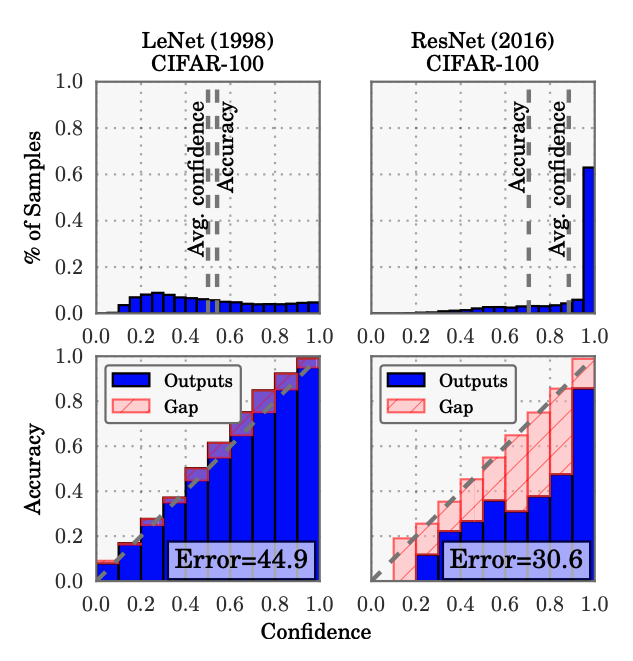• 假设“与预测的类标签相关的概率应该反映其真实正确性的可能性。”
• 现实“现代（大型）神经网络不再经过良好校准”
• 解决方案：在模型输出上应用temperature scaling（普拉特缩放的扩展）

pip install cleanlab==1.0.1 -q

import cleanlab
from cleanlab.pruning import get_noise_indices

# Determine potential labeling errors
label_error_indices = get_noise_indices(
s=y_test,
psx=y_prob,
sorted_index_method="self_confidence",
verbose=0)


num_samples = 5
for index in label_error_indices[:num_samples]:
print ("text:", test_df.iloc[index].text)
print ("true:", test_df.iloc[index].tag)
print ("pred:", label_encoder.decode([y_pred[index]]))
print ()

text: module 2 convolutional neural networks cs231n lecture 5 move fully connected neural networks convolutional neural networks
true: computer-vision
pred: other


## Manual slices手动切片

• 目标/预测类别（+ 组合）
• 特征（显式和隐式）
• 元数据（时间戳、来源等）
• Priority slices/experience（少数群体、大客户等）

pip install snorkel==0.9.8 -q

from snorkel.slicing import PandasSFApplier
from snorkel.slicing import slice_dataframe
from snorkel.slicing import slicing_function

@slicing_function()
def nlp_cnn(x):
"""NLP Projects that use convolution."""
nlp_projects = "natural-language-processing" in x.tag
convolution_projects = "CNN" in x.text or "convolution" in x.text
return (nlp_projects and convolution_projects)


nlp_cnn_df = slice_dataframe(test_df, nlp_cnn)

text    tags
126    understanding convolutional neural networks nl...    natural-language-processing

short_text_df = slice_dataframe(test_df, short_text)

    text    tags
44    flashtext extract keywords sentence replace ke...    natural-language-processing
62    tudatasets collection benchmark datasets graph...    other
70    tsfresh automatic extraction relevant features...    other
88    axcell automatic extraction results machine le...    computer-vision


# Slices
slicing_functions = [nlp_cnn, short_text]
applier = PandasSFApplier(slicing_functions)
slices = applier.apply(test_df)
slices

rec.array([(0, 0), (0, 0), (0, 0), (0, 0), (0, 0), (0, 0), (0, 0), (0, 0),
(1, 0) (0, 0) (0, 1) (0, 0) (0, 0) (1, 0) (0, 0) (0, 0) (0, 1) (0, 0)
...
(0, 0), (0, 0), (0, 0), (0, 0), (0, 0), (0, 0), (0, 0), (0, 1),
(0, 0), (0, 0)],
dtype=[('nlp_cnn', '<i8'), ('short_text', '<i8')])


# Score slices
metrics["slices"] = {}
for slice_name in slices.dtype.names:
slice_metrics = precision_recall_fscore_support(
)
metrics["slices"][slice_name] = {}
metrics["slices"][slice_name]["precision"] = slice_metrics
metrics["slices"][slice_name]["recall"] = slice_metrics
metrics["slices"][slice_name]["f1"] = slice_metrics

print(json.dumps(metrics["slices"], indent=2))

{
"nlp_cnn": {
"precision": 1.0,
"recall": 1.0,
"f1": 1.0,
"num_samples": 1
},
"short_text": {
"precision": 0.8,
"recall": 0.8,
"f1": 0.8000000000000002,
"num_samples": 5
}
}


• 足够的数据（新的或过采样的不正确预测）
• 屏蔽算法（使用文本匹配启发式）

## Generated slices生成的切片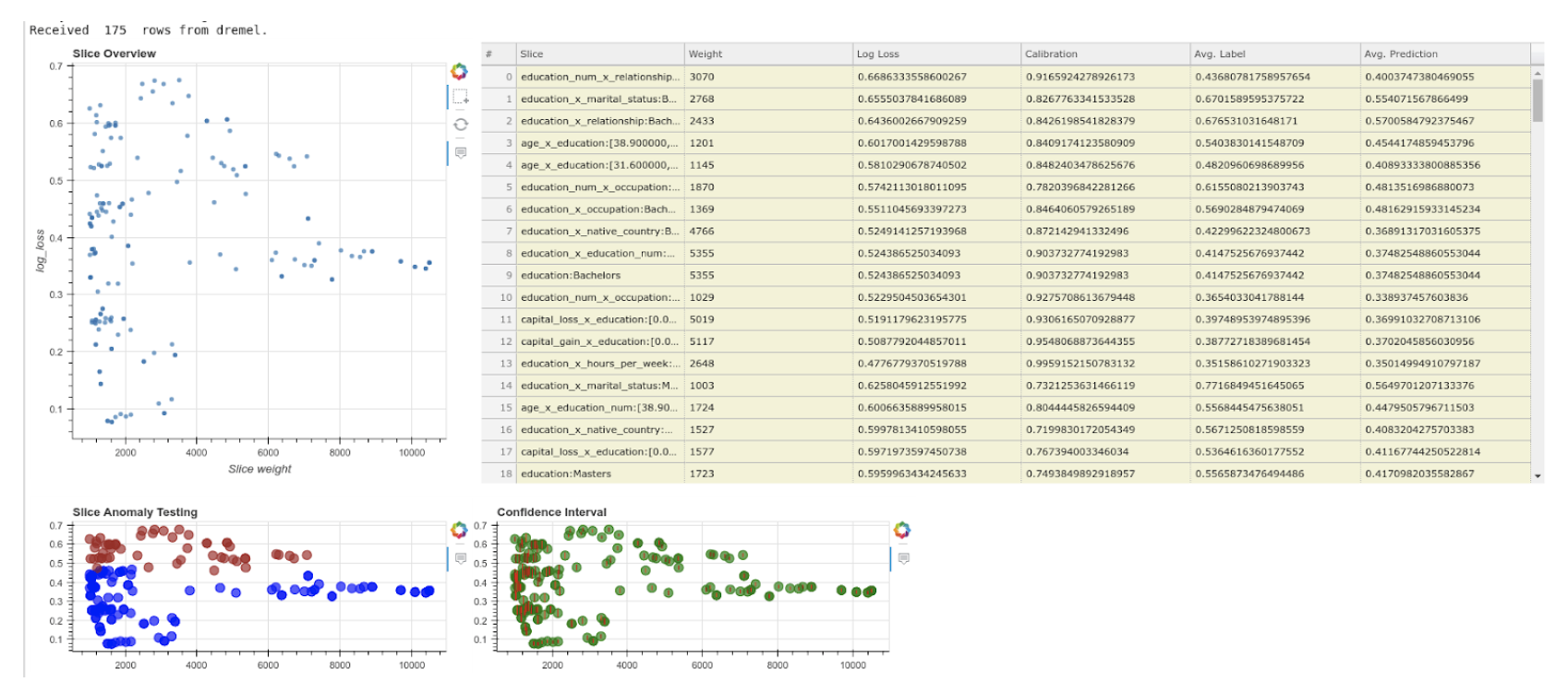### 隐藏分层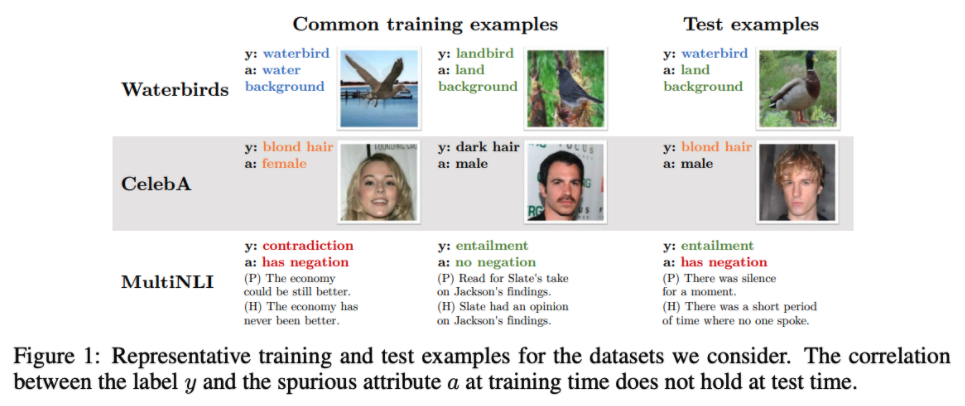1. 通过无监督聚类估计隐式子类标签
2. 使用这些集群训练新的更强大的模型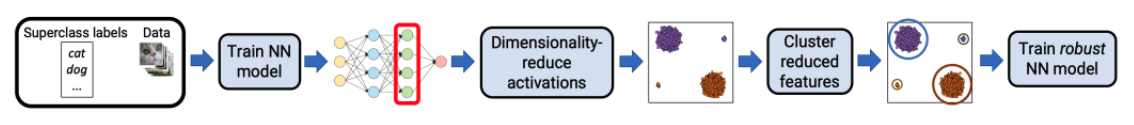### 模型修补

1. 学习小组
2. 学习在同一超类（标签）下从一个子组到另一个子组所需的转换（例如CycleGAN
3. 使用人为引入的子组特征来增强数据
4. 在增强数据上训练新的稳健模型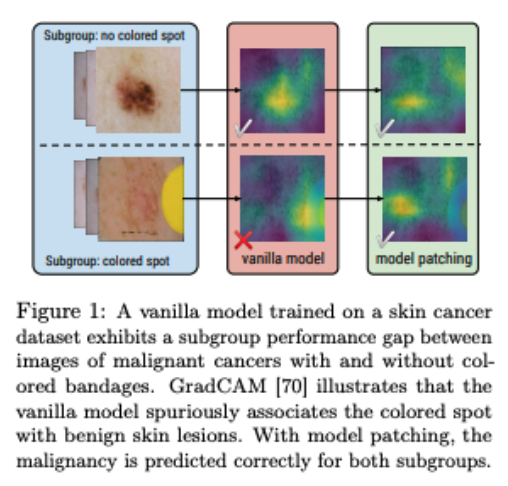## 可解释性

pip install lime==0.2.0.1 -q

from lime.lime_text import LimeTextExplainer
from sklearn.pipeline import make_pipeline


# Create pipeline
pipe = make_pipeline(vectorizer, model)

# Explain instance
text = "Using pretrained convolutional neural networks for object detection."
explainer = LimeTextExplainer(class_names=label_encoder.classes)
explainer.explain_instance(text, classifier_fn=pipe.predict_proba, top_labels=1).show_in_notebook(text=True)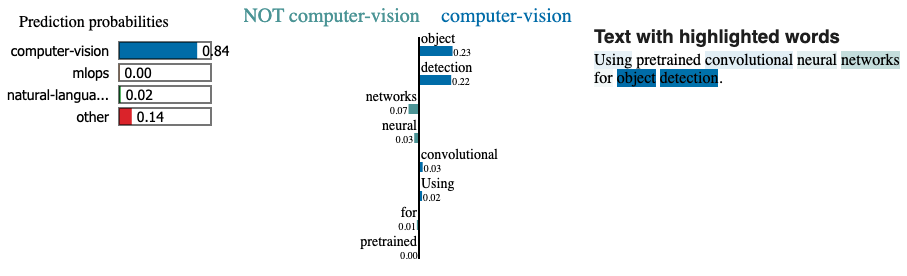## 反事实## 行为测试

• invariance：更改不应影响输出。
# INVariance via verb injection (changes should not affect outputs)
tokens = ["revolutionized", "disrupted"]
texts = [f"Transformers applied to NLP have {token} the ML field." for token in tokens]
predict_tag(texts=texts)

['natural-language-processing', 'natural-language-processing']

• directional：变化应该会影响产出。
# DIRectional expectations (changes with known outputs)
tokens = ["text classification", "image classification"]
texts = [f"ML applied to {token}." for token in tokens]
predict_tag(texts=texts)

['natural-language-processing', 'computer-vision']

• minimum functionality：输入和预期输出的简单组合。
# Minimum Functionality Tests (simple input/output pairs)
tokens = ["natural language processing", "mlops"]
texts = [f"{token} is the next big wave in machine learning." for token in tokens]
predict_tag(texts=texts)

['natural-language-processing'，'mlops']


• 什么标准最重要？
• 哪些标准可以/不能回归？
• 可以容忍多少回归？

## 在线评估

• 手动标记传入数据的子集以定期评估。
• 询问查看新分类内容的初始用户组是否正确分类。
• 允许用户通过模型报告错误分类的内容。

### AB 测试

AB 测试涉及将生产流量发送到当前的系统（控制组）和新版本（处理组），并测量两个指标的值之间是否存在统计差异。AB 测试有几个常见问题，例如考虑不同的偏差来源，例如向某些用户展示新系统的新颖性效果。还需要确保相同的用户继续与相同的系统进行交互，以便可以比较结果而不会造成污染。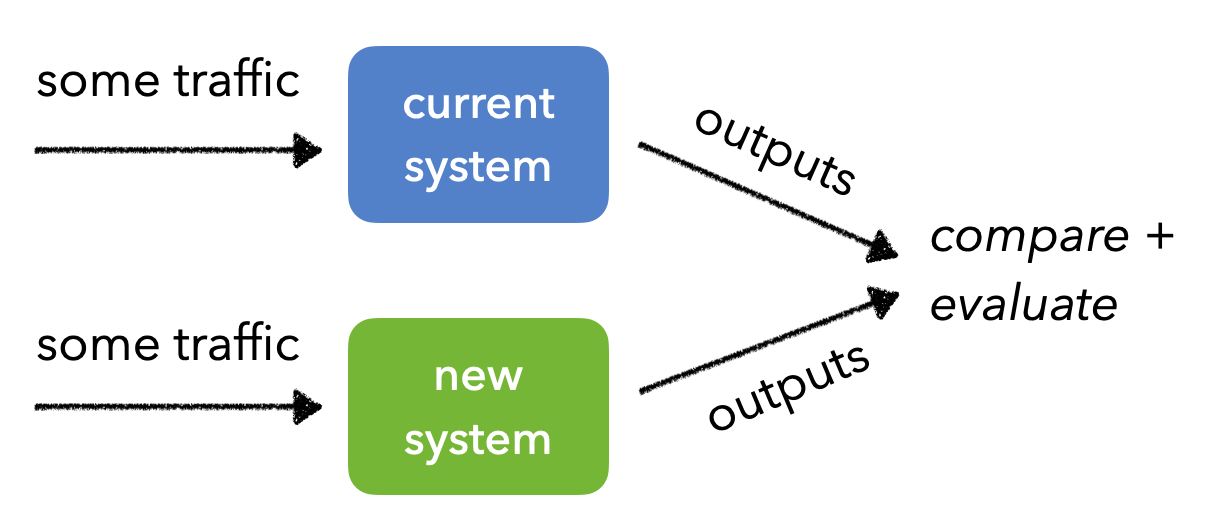### canary测试

Canary 测试涉及将大部分生产流量发送到当前部署的系统，但将一小部分用户的流量发送到正在尝试评估的新系统。同样，随着逐步推出新系统，需要确保相同的用户继续与相同的系统进行交互。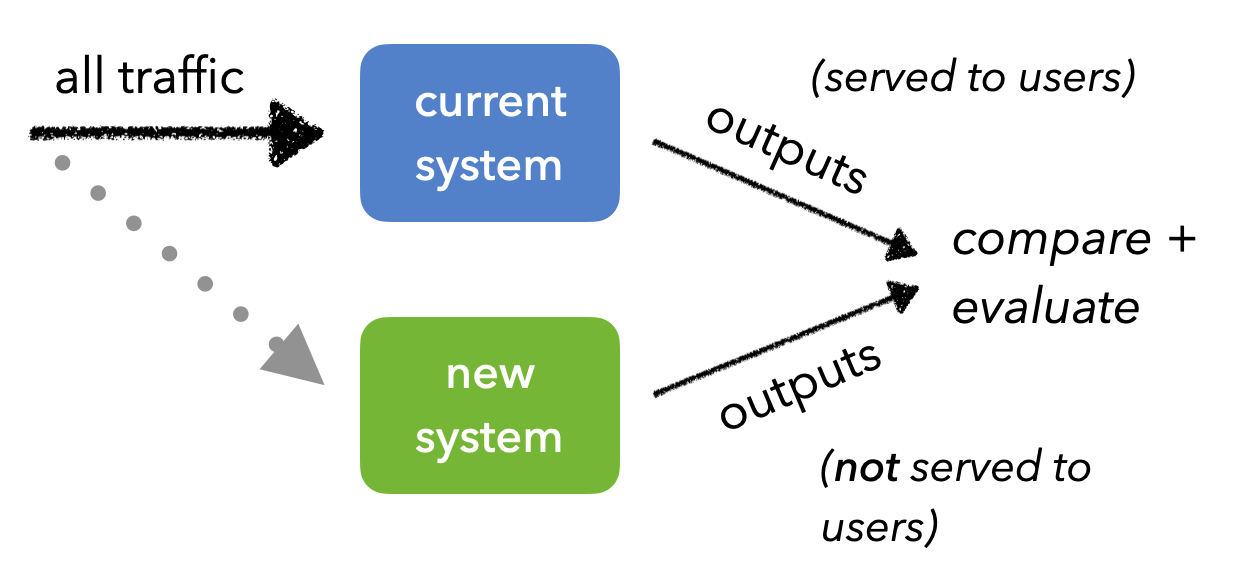A/B 测试：统计测试的完整指南

## Resources@article{madewithml,
author       = {Goku Mohandas},
title        = { Made With ML },